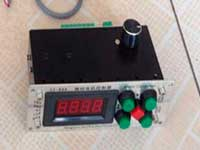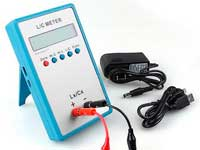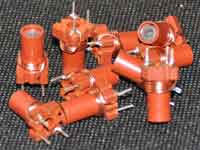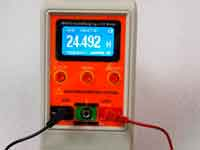Rating 0.00 (0 Votes)

Online calculators

Inductance as a straight wire over the plain with one grounded end:

Inductance is used on UHF. Of course, we have to present this inductor as a segment of the transmission line to get the more right result. However, this formula has a reasonable accuracy.

The calculation formula is taken from the ARRL Handbook For Radio Communications:• L = Inductance µH
• a = radius of the wire in inches
• b = length of the conductor parallel to the plain in inches
• h = height above the plain in inchesProposed below calculator uses this formula with sizes transformed in the metric system:

ENTER THE INPUT DATA:
 b = mmcm – The length of the conductor h =  mm – The height of the conductor d =  mm – The diameter of the wire

RESULT:
 L = nHμH – Inductance

Another similar calculators:

Сomments from anonymous guests are enabled with moderation.

Coil Winding MachineUS \$187.53
The system consists of individual components including a controller, a stepping motor, a foot switch, power adapter and output shaft. This design makes it very easy for you to integrate the system into your own project.

LC Meter LC200AUS \$37.99
Measuring Capacitance Range:
0.01 pF - 100mF
Measuring Inductance Range:
0.001 uH - 100H
Measurement accuracy:1%.

RF InductorsUS \$6.43
Variable Ferrite Core Inductor coil set 3.5Turns 25-100MHZ

Auto Ranging LCR MeterUS \$63.07
Meter can measure 0.00pF to 100.00mF capacitance, 0.000uH to 100.00H inductance and 0.00? to 10.000M? resistance. Very suitable for small value capacitor and inductor measurement.